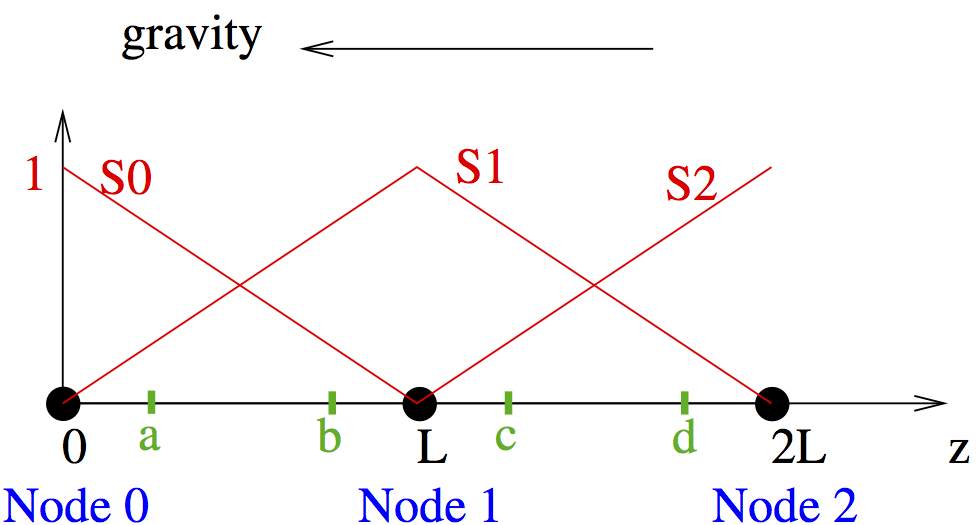# Mass lumping

This page is part of a set of pages devoted to discussions of numerical stabilization in PorousFlow. See:

Consider here just the fluid-flow equation, as the heat-energy equation is analogous. The time-derivative term is discretised as

(1)

Here is the test function that we are integrating against. An alternative discretisation would be (in the single-phase situation), but Eq. (1) conserves mass more effectively than other alternatives.

In the standard finite-element scheme, and its individual parts (porosity, saturation, etc) are evaluated at each quadrature point. However, in PorousFlow, everything in the time derivative is evaluated at the nodes. Specifically, at a node depends only on the independent variables at that node. It has been shown in many studies that this lumping is advantageous for mass conservation and reduces spurious oscillations of the pressure around sharp fronts (Celia et al., 1990).

The cause of oscillations around sharp fronts, and how mass lumping removes the oscillations, can be illustrated through a simple example.Figure 1: Two elements of length . Linear Lagrange shape/test functions for each node are shown in red ( for node 0, for node 1, and for node 2). Gravity acts in the direction . Gauss points are shown in green.

Consider the situation in Figure 1, and suppose that Node 2 has high potential, and that Nodes 0 and 1 are at residual saturation where the relative permeability is zero. Then fluid will flow from Node 2 to Node 1 (and then to Node 0 in the next time step). For simplicity, imagine that the fluid mass, , is a linear function of the potential. Then, up to constants, the discretised mass-conservation equation without mass lumping reads

(2)

The matrix on the LHS comes from performing the numerical integration of over the two elements. Note that it is not diagonal because the integration over an element depends on the mass at both of its two nodes. The RHS encodes that no fluid is flowing between Nodes 0 and 1, but fluid is flowing from Node 2 to Node 1.

The important point is the solution of these sets of equations is (3) This means the finite element solution of the mass-conservation equation will be oscillatory around fronts.

However, with mass lumping, the matrix in the above equation becomes diagonal, and the solution is . Explicitly, the contribution to the residual at node is

(4) where is evaluated at node using the independent (nonlinear) variables evaluated at that node, and qp are the quadpoints.

There is one small complication. Porosity may be dependent on volumetric strain, which is dependent on the gradients of the displacement variables, which are evaluated at the quadpoints, not the nodes. In this case, the porosity at node is assumed to be dependent on the volumetric strain evaluated at the closest quadpoint to the node.

1. M.A. Celia, E. T. Bouloutas, and R. L. Zabra. A general mass-conservative numerical solution for the unsaturated flow equation. Water Resources Research, 26:1483–1496, 1990.[BibTeX]